# SAS/STAT Examples

## Fitting a Mixture of Exponential Distributions for Patient’s Length of Stay

Contents | SAS Program | PDF

## Overview

Health service researchers frequently study length of hospital stay (LOS) as a health outcome. Generally originating from heavily skewed distributions, LOS data can be difficult to model with a single parametric model. Mixture models can be quite effective in dealing with such data. This example illustrates how to perform a Bayesian analysis of an exponential mixture model for LOS data. The experimental MCMC procedure is used for this analysis.

## Analysis

The LOS data analyzed in this example originate from geriatric patients in a psychiatric hospital in North East London in 1991 and were studied by Harrison and Millard ( 1991 ) and McClean and Millard ( 1993 ) . Each observation represents the LOS in days for an admitted patient.

```   data inputdata;
input los @@;
datalines;
1671 1300   722   586   552   525  364
359  321   302   272   248   226  216
208  182   141   141   132   120  117
115  114   113   104   103   101   99
96   94    93    92    88    84   83
81   79    74    70    63    62   62
61   56    55    53    53    51   51
50   49    36    33    33    33   29
28   26    24    19    16    16   15

... more lines ...

35   28    20    19     9     5    2
2    2 22317 14006 11549 11006 8981
8402 7947  7266  6693  4408  4010 4003
1970 1857  1849  1833  1770  1769 1514
1217  956   924   611   386   280   93
;
```

Before using this data in a mixture model setting, use the MEANS procedure to obtain summary statistics for the LOS data in table form. Figure 1 displays summary statistics for this data.

Figure 1 PROC MEANS Summary of LOS
The MEANS Procedure

Analysis Variable : los
N Mean Std Dev Minimum Maximum
469 3712.36 5675.97 1.0000000 24028.00

Figure 2 displays a plot that illustrates the skewness of the data. The histogram of the data is overlaid with a scaled exponential density, and you can see that a single exponential density does not fit the lower values of LOS well.

Figure 2 Length of Stay, Exponential Density## Bayesian Mixture of Exponential Model

Mixture models arise naturally when one mechanism generates data according to one model and another mechanism generates data according to a different model. In this data set, the variable that indicates which mechanism generated an observation is not recorded, and only the response variable is available. A mixture model is useful for analyzing this data set because it unveils the latent heterogeneity that arises from a latent categorical variable (Fruhwirth-Schnatter; 2006 ) .

Mixture models can be expressed as a weighted average ofcomponent densities. More formally,
is said to arise from a finite mixture model with probability density function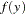where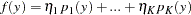For all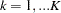,is the component probability density function, and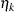are the weights defined by the following constraints:and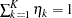.

Congdon ( 2003 ) states that exponential mixture models with relatively small number of components are effective in modeling skewed LOS data. You can write a two-component exponential mixture model for LOS data with density as follows: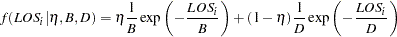for patients.

There are three parameters in the density:, and. Researchers Harrison and Millard ( 1991 ) suggest the mean parameters,and, as the average LOS for the standard- and long-stay groups, respectively. In addition,represents an unknown fraction of patients in the standard-stay group with.

Suppose the following prior distributions are placed on the three parameters: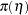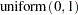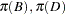where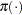indicates a prior distribution and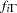is the density function for the inverse-gamma distribution. Priors of this type are often called diffuse priors . Theprior expresses your lack of knowledge about the mixture proportion.

Label-switching is a common problem that arises in mixture models. It was described by as a result of the invariance of the mixture likelihood function under the relabeling of the mixture components. In an effort to remove label-switching, Harrison and Millard ( 1991 ) place an identifiability constraint that the average LOS of the standard-stay group is less than that of the long-stay group; that is,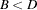.

Using Bayes’ theorem, the likelihood function and prior distributions determine the posterior distribution of, andas follows: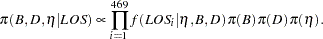PROC MCMC obtains samples from the desired posterior distribution, which is determined by the prior and likelihood specified. It does not require the form of the posterior distribution.

The following SAS statements use the prior distributions to fit the Bayesian exponential mixture model. The PROC MCMC statement invokes the procedure and specifies the input data set. The NMC= option specifies the number of posterior simulation iterations. The THIN=5 option specifies that one of every five samples is kept. The PROPCOV=QUANEW option uses the estimated inverse Hessian matrix as the initial proposal covariance matrix.

```   ods graphics on;
proc mcmc data=inputdata seed=1010 nmc=50000 thin=5
propcov=quanew;
ods output PostSummaries = post_summ;
parms B 100 D 6000 pi 0.5;
prior B D ~ igamma(3/10, scale = 10/3);
prior pi ~ uniform(0,1);
if (B < D) then
llike = log(pi*pdf("expo", los, B) + (1-pi)*pdf("expo", los, D));
else
llike = .;
model general(llike);
run;
ods graphics off;
```

The ODS OUTPUT statement creates an output data set post_summ used later for a graphical analysis of the fit in Figure 8 . The PARMS statement puts all three parameters,, andin a single block and assigns initial values to each of them. The PRIOR statements specify priors for all the parameters. Note thatandcan be specified with one PRIOR statement because they have the same prior distribution.

The IF-ELSE statements enable different values of LOS to have different log-likelihood functions, depending on whether the order constraint placed on the mean parameters is satisfied. The MODEL statement specifies that llike is the log likelihood for each observation in the model and is simply a missing value when the order constraint is not met.

By turning ODS Graphics on, PROC MCMC produces graphs at the end of the procedure which enable you to visually examine the convergence of the chain. See Figure 3 . Inferences should not be made if the Markov chain has not converged.

Figure 3 LOS Diagnostic Plots for,, and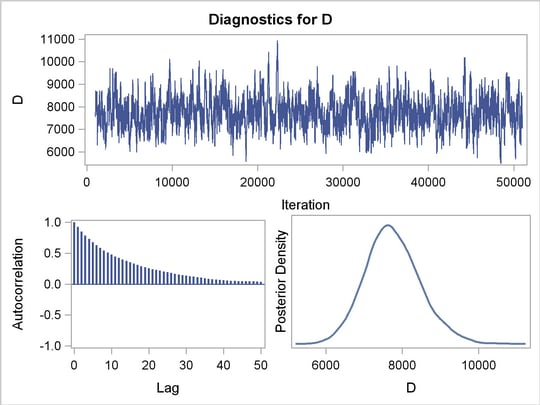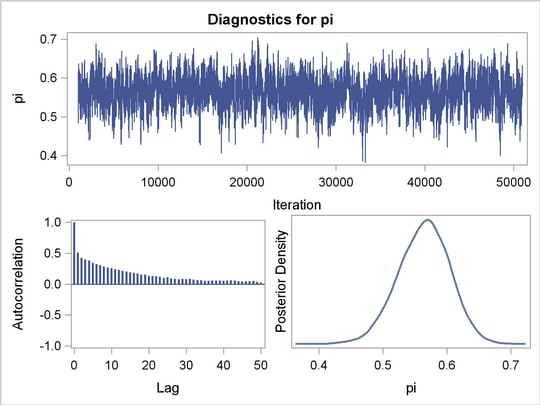Figure 3 displays convergence diagnostic graphs for parameters,, and. The trace plots indicate that the chains appear to have reached stationary distributions. The chain also has good mixing and is dense.

The autocorrelation plots indicate low autocorrelation and efficient sampling. Finally, the kernel density plots show the smooth, unimodal shape of posterior marginal distributions for each parameter. If label-switching had occured, you would see jumps in the trace plots or multimodal density plots.

Figure 4 contains the "Parameters" table which lists the names of the parameters, the blocking information, the sampling method used, the starting values, and the prior distributions.

Figure 4 LOS Parameter Information
Parameters
Parameter Sampling
Method
Initial
Value
Prior Distribution
B N-Metropolis 100.0 igamma(3/10, scale = 10/3)
D N-Metropolis 6000.0 igamma(3/10, scale = 10/3)
pi N-Metropolis 0.5000 uniform(0,1)

The "Tuning History" table, shown in Figure 5 , displays how the tuning stage progresses for the multivariate random walk Metropolis algorithm used by PROC MCMC to generate samples from the posterior distribution. An important aspect of the algorithm is the calibration of the proposal distribution. The tuning of the Markov chain is broken into a number of phases. In each phase, PROC MCMC generates trial samples and automatically modifies the proposal distribution as a result of the acceptance rate.

The "Burn-In History" and the "Sampling History" tables show the burn-in and main phase sampling, respectively.

Figure 5 LOS Burn-In and Sampling History
Tuning History
Phase Block Scale Acceptance
Rate
1 1 2.3800 0.0280
2 1 1.0123 0.0700
3 1 0.5221 0.3420

Burn-In History
Block Scale Acceptance
Rate
1 0.5221 0.3980

Sampling History
Block Scale Acceptance
Rate
1 0.5221 0.3811

Figure 6 displays summary and interval statistics for each posterior distribution.

Figure 6 LOS MCMC Summary and Interval Statistics
The MCMC Procedure

Posterior Summaries
Parameter N Mean Standard
Deviation
Percentiles
25% 50% 75%
B 10000 619.1 74.2261 568.9 618.3 668.3
D 10000 7752.6 721.2 7267.1 7710.4 8202.1
pi 10000 0.5638 0.0403 0.5370 0.5655 0.5916

Posterior Intervals
Parameter Alpha Equal-Tail Interval HPD Interval
B 0.050 473.6 767.0 472.0 763.7
D 0.050 6418.1 9288.4 6339.5 9195.0
pi 0.050 0.4826 0.6389 0.4825 0.6387

Figure 7 reports a number of convergence diagnostics to assist in determining convergence. These are the Monte Carlo standard errors, the autocorrelations at selected lags, the Geweke diagnostics, and the effective sample sizes.

Figure 7 LOS MCMC Convergence Diagnostics
The MCMC Procedure

Monte Carlo Standard Errors
Parameter MCSE Standard
Deviation
MCSE/SD
B 3.9598 74.2261 0.0533
D 38.2006 721.2 0.0530
pi 0.00164 0.0403 0.0408

Posterior Autocorrelations
Parameter Lag 1 Lag 5 Lag 10 Lag 50
B 0.9150 0.6588 0.4459 0.0689
D 0.9220 0.6766 0.4760 0.0354
pi 0.5086 0.3438 0.2564 0.0267

Geweke Diagnostics
Parameter z Pr > |z|
B 0.1718 0.8636
D -0.2669 0.7895
pi -0.3073 0.7586

Effective Sample Sizes
Parameter ESS Correlation
Time
Efficiency
B 351.4 28.4595 0.0351
D 356.4 28.0580 0.0356
pi 601.9 16.6129 0.0602

Figure 6 displays the marginal posterior summaries. The larger group of standard-stay patients has an average LOS ofdays relative to the smaller group of long-stay patients whose stay averages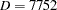days. The posterior average mixture proportions are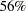and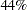for the standard-stay and long-stay group, respectively.

Figure 8 illustrates the scaled mixture of exponential density and the gain in model fit from the two-component exponential mixture model.

Figure 8 Length of Stay, Exponential and Mixture DensityThe single-component exponential model had an average LOS of 3712 days. The mean parameters found when fitting an exponential mixture model to the standard-stay group and the long-stay group are 619 and 7752 days, respectively. The mixture distribution fits the data better than the exponential distribution, especially at the low values of LOS. Additional components could be fit and evaluated in similar methods or with information criteria.

## References

Congdon, P. (2003), Applied Bayesian Modeling , John Wiley & Sons.

Fruhwirth-Schnatter, S. (2006), Finite Mixture and Markov Switching Models , Springer.

Harrison, G. and Millard, P. (1991), “Balancing Acute and Long-Term Care: The Mathematics of Throughput in Departments of Geriatric Medicine,” Meth. Infor. Medicine , 30, 221–228.

McClean, S. and Millard, P. (1993), “Patterns of Length of Stay after Admission in Geriatric Medicine: An Event History Approach,” The Statistician , 42(3), 263–274.